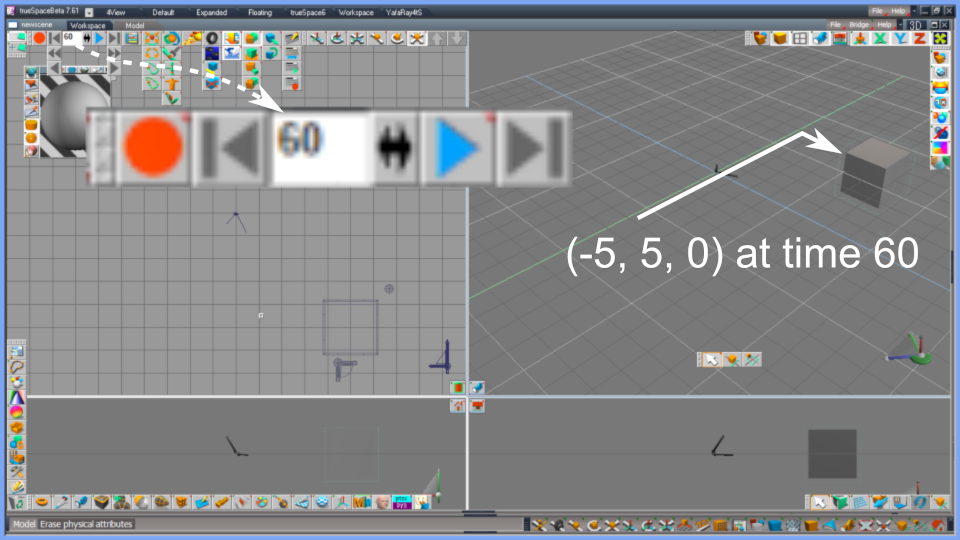## 0100. Animation

Getting started, ptsxpy, Python language, trueSpace, programming, syntax highlighting for text editor, etc.
Note: it's provisional and topics will be added and revised at random till some sort of documentations are prepared.
3DfromNULL
Posts: 45
Joined: 2018-Jul-13 9:35

### 0100. Animation

To make an animation of an object in trueSpace (tS) using ptsxpy, we make "time frames" of the object at each of multiple "active time". The code below creates a cube, makes 3 frames of it, and changes its position per each frame.

Code: Select all

import ptsxpy as p

cb1 = p.CreateCube( 1, 2., 2., 2. )

tm = 0.
dt = 30.

p.AnimSetActiveTime( tm )
p.SobjSetFrame( cb1, e_tsxKFT_MOVE )
loc1 = Vec3f( 5., 0., 0. )
p.GNodeSetLocation( cb1, loc1.p )

tm += dt
p.AnimSetActiveTime( tm )
p.SobjSetFrame( cb1, e_tsxKFT_MOVE )
loc1 = Vec3f( -5., 0., 0. )
p.GNodeSetLocation( cb1, loc1.p )

tm += dt
p.AnimSetActiveTime( tm )
p.SobjSetFrame( cb1, e_tsxKFT_MOVE )
loc1 = Vec3f( -5., 5., 0. )
p.GNodeSetLocation( cb1, loc1.p )

p.SceneDraw()

1. Please make sure where the "Play" button os tS is. It's a triangle-shaped arrow, pointing right. Red for tS4, tS5, Blue for tS6.6 (model side of tS7).
2. Execute the script above using ptsxpy.
3. Make sure that the "Play Mode" of trueSpace is "Scene" (you can check that by right-clicking "Play" button).
4. Click "Play" button to start the animation made by the script.

The generated cube moves as a result. The images below is at each time frame. The trueSpace interpolates between each frame automatically, and the cube moves smoothly.Fig.1. Position at time 0Fig.2. Position at time 30Fig.3. Position at time 60

We can visually check the generated time sequence using the Scene Editor (Key Frame Editor, KFE for tS4, tS5) of trueSpace.Fig.4. The Scene Editor (Key Frame Editor, KFE for tS4, tS5)

The three time frames are indicated by rhombus icons in the "Move" line. The code which made it "Move" is not GNodeSetLocation() but e_tsxKFT_MOVE.

Code: Select all

p.SobjSetFrame( cb1, e_tsxKFT_MOVE )

If mistakenly you combined e_tsxKFT_ROTATE with GNodeSetLocation(), you should not be able to get any expected animation. Please note that it's necessary to keep consistency between the 2nd parameter of SobjSetFrame() and other actions to the object like moving, rotating, scaling, deforming, color-changing, etc.

You can specify multiple SobjSetFrame() and do corresponding multiple actions to an object in order to get an animation where multiple actions composite. You can also make layered animations on tS using a group object. In the sample script "Fan (2019)", an electric fan lengthens its neck, then rotates its fan blades and shakes its head in the same time. The fan animation might be able to made easier by hand. Scripting is more suitable/effective for scenes in which more larger number of objects move systematically like domino toppling.

Scripts can also generate time frame for other object than moving of polyhedrons; vertices, cameras, lights, textures, bump (displacement) maps, colors, etc. The ptsxpydef1.py defines a set of types for SobjSetFrame() according to a C++ enum defined in tsxAPIxx.doc (and SDK header files). It includes if-statements because the number of enum elements is differ between tS version as below;

Code: Select all

#---------------------
#  enum tsxKFRAME_TYPE
#---------------------
e_tsxKFT_UNDEF       =  0
e_tsxKFT_LOOK        =  1
e_tsxKFT_ROTATE      =  2
e_tsxKFT_MOVE        =  3
e_tsxKFT_SCALE       =  4
e_tsxKFT_DEFORM      =  5
e_tsxKFT_RECT        =  6
e_tsxKFT_COLOR       =  7
e_tsxKFT_SURFACE     =  8
e_tsxKFT_BUMP        =  9
e_tsxKFT_TEXTURE     = 10
e_tsxKFT_PROCTEX     = 11
e_tsxKFT_ENVIRON     = 12
e_tsxKFT_FACETCOS    = 13
e_tsxKFT_LCOLOR      = 14
e_tsxKFT_BACKGROUND  = 15
e_tsxKFT_GLOBENV     = 16
e_tsxKFT_FOG         = 17
e_tsxKFT_RAYTRACE    = 18
e_tsxKFT_PLUGIN      = 19
e_tsxKFT_NAIL        = 20
e_tsxKFT_JOINT       = 21
e_tsxKFT_KINEMATICS  = 22
e_tsxKFT_VERTEX      = 23
e_tsxKFT_INVISIBLE   = 24
e_tsxKFT_SWEEP       = 26
if tscode <= 43:      # tS4.3
e_tsxKFT_END       = 27
else:
e_tsxKFT_NURBTENS    = 27
e_tsxKFT_NURBSPATCH  = 28
e_tsxKFT_NURBSCURVE  = 29
e_tsxKFT_SUBDIVISION = 30
e_tsxKFT_MB_MUSCLE   = 31
if tscode <= 60:    # tS5.0 - tS6.0
e_tsxKFT_END       = 32
else:                 # tS6.6 - tS7
e_tsxKFT_PH_ACCMOV   = 32
e_tsxKFT_PH_ACCROT   = 33
e_tsxKFT_PH_COARSE   = 35
e_tsxKFT_PH_ELAST    = 36
e_tsxKFT_PH_ERESIST  = 37
e_tsxKFT_PH_FRICT    = 38
e_tsxKFT_PH_CHARGE   = 39
e_tsxKFT_PH_INITMOV  = 40
e_tsxKFT_PH_INITROT  = 41
e_tsxKFT_PH_WEIGHT   = 42
e_tsxKFT_PH_ACTFLG   = 43
e_tsxKFT_PHE_GRAVC   = 44
e_tsxKFT_PHE_ATM     = 45
e_tsxKFT_PH_CG       = 46
e_tsxKFT_END         = 47

As you can see, tS4 does not have the elements for NURBS and metaball muscle, tS5 and tS6.0 have them but don't have ones for physics simulation, and tS6.6 has all. We cannot make any animation on tS using e_tsxKFT_xxxx that is not defined for each tS version.

The animation played by the "Play" button of tS is some sort of preview. After you rendered it to a file, you can watch it using your movie player in right time sequence. In case you render it with 30 fps (frames per second), the duration (time length of playing time) of the animation is ( 60 / 30 ) = 2 seconds in this example. The cube moves faster in the first 1 second than the last 1 second because the distance is longer than the last 1 second in case of this code.

Please note that the parameter of AnimSetActiveTime() must be a floating point value as the code above.